# Linear regression with quadratic equations

Linear regression is the technique by which we mathematically find a “line of best fit” (which is not necessarily a straight line) for a particular set of data. This technique is widely used in science, engineering, business, research, and more; in order to find relationships between different variables and make predictions about their future behaviour. In my previous article “Linear regression with straight lines”, we looked at the mathematics of fitting a straight line to a data set. The limitations of doing this are clear: not every relationship between a set of variables is linear (in fact, most relationships aren’t). For this reason, we will now extend our analysis to quadratic equations (e.g. parabolas).

# Mathematical derivation of the optimum parameters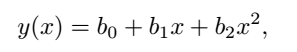Equation 1: equation of best “fit”

In this case, there are 3 parameters we must solve for, so as we might expect this will require us to solve a 3x3 matrix equation. First, we write the sum-squared error function as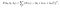Equation 2: sum squared error

To create 3 equations, we will take 3 partial derivatives, one with respect to each parameter b0, b1 and b2

and equating all of them to zero in order to find the turning points yields

And upon making the substitution

we obtain the solution for the parameters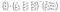Equation 3: solution to the parameters b0, b1 and b2

Another important quantity that we ought to calculate is the R² value. Known as the “coefficient of determination”, it gives us a measure of how closely the fit describes the data. A value of R² = 1 means that the fit perfectly describes 100% of the data, while an R² = 0 means the fit doesn’t describe any of the data. In practice, we tend to select something close to 1 as our minimum margin of acceptance, for example R² = 0.95 is used in some sub-fields of science as it roughly suggests that “95% of the variability in the data S(x) is explained by changes in the dependent variable x”. The R² value is calculated from the equation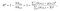Equation 4: R² value

where

is the mean or average value of the entire data-set S(x).

# Implementation in MATLAB

%%%%%%%%%%%%%%%%%%%%%%%%%%%%%%%%%%%%%%%

% Least-Squares-Analysis (LSA) for quadratic fit

%

% Description: generates a random data-set around a quadratic curve with

% preset values and then uses LSA matrix methods to find the coefficients

% of the line of best-fit, and overlays the fit-line to the dataset:

% Equation → y = b0 + b1*x + b2*x²

%

% Made by: Oscar A. Nieves

% Made in: 2019

%%%%%%%%%%%%%%%%%%%%%%%%%%%%%%%%%%%%%%%

clear all; close all; clc;

% Generate dataset

x = 0:0.1:5;

S = 3*x.² — x + 5*randn(size(x));

%% Quadratic LSA

n = length(S);

sum_x = sum(x);

sum_x2 = sum(x.²);

sum_x3 = sum(x.³);

sum_x4 = sum(x.⁴);

sum_S = sum(S);

sum_xS = sum(x.*S);

sum_x2S = sum(x.².*S);

A = [ [n, sum_x, sum_x2]; …

[sum_x, sum_x2, sum_x3];…

[sum_x2, sum_x3, sum_x4] ];

RHS = [sum_S, sum_xS, sum_x2S].’;

b = A\RHS;

b0 = b(1);

b1 = b(2);

b2 = b(3);

% Fit parabola to data

y = b0 + b1*x + b2*x.²;

% Calculate R² coefficient of determination

R2_quad = sum( (y — mean(S)).² )./sum( (S — mean(S)).² );

disp([‘R² = ‘ num2str(R2_quad)]);

% Plots results

figure(1);

set(gcf,’color’,’w’);

scatter(x,S); hold on;

plot(x,y,’r’,’LineWidth’,3); hold off;

legend(‘Raw Data’,’Fit-line’); legend boxoff;

legend(‘Location’,’northwest’);

title([‘b_0 = ‘ num2str(round(b0,2)) ‘, b_1 = ‘ num2str(round(b1,2)) …

‘, b_2 = ‘ num2str(round(b2,2))]);

xlabel(‘x’);

ylabel(‘y’);

axis tight;

set(gca,’FontSize’,20);

%%%%%%%%%%%%%%%%%%%%%%%%%%%%%%%%%%%%%%%

A single run of this code produces the following plot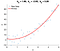Figure 1: quadratic least squares analysis of a random data set

with a coefficient of determination of R² = 0.93919.

Oscar is a physicist, educator and STEM enthusiast. He is currently finishing a PhD in Theoretical Physics with a focus on photonics and stochastic dynamics.

## More from Oscar Nieves

Oscar is a physicist, educator and STEM enthusiast. He is currently finishing a PhD in Theoretical Physics with a focus on photonics and stochastic dynamics.